Related Articles

# Asynchronous Down Counter

• Last Updated : 15 Jun, 2021

Counter :
Counters are used to count the clock pulses. The clock pulses occur at regular intervals. They are used to measure the  time and frequency. We can also say that counters are sequential circuits which change their pre-defined states with the help of clock pulses. They are constructed with flip flops and logic gates.

They are of two types –

1. Asynchronous counter
2. Synchronous counter

In the asynchronous counter, an external clock pulse is provided for only the first flip flop, thereafter the output of the 1st FF acts as a clock pulse for the second FF and so on. In the case of synchronous FFs, all the flip flops are triggered simultaneously by an external clock pulse.
Please refer this, to understand how an asynchronous counter works.

3 bit asynchronous down counter :

• For the 3 bit counter, we require 3 flip flops and we can generate 23 = 8 state and count(111 110 … 000).
• We can generate down counting states in an asynchronous down counter by two ways.

Method 1 :
In this implementation, the clock pulse(of 50% duty cycle) is given to only the first FF. Thereafter, the output of the first FF is feed as a clock to second FF and the output of the second FF is feed as the clock for the third FF. But the complemented output is taken from each FF(i.e. same as Up counter but output states are complemented). Here QA is LSB and QC is MSB.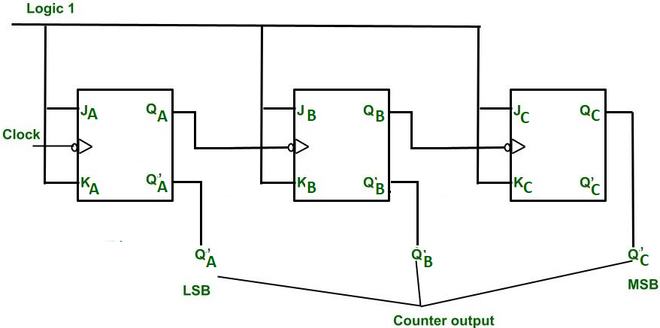State table –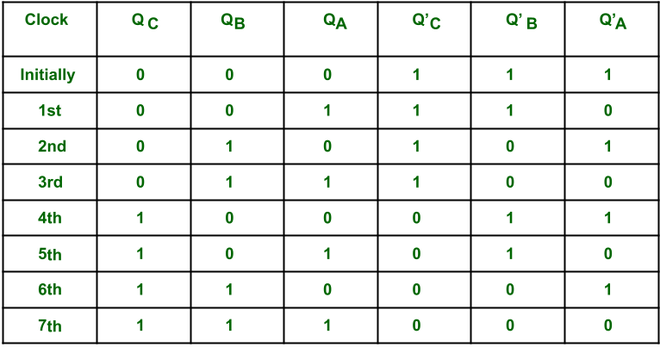Method 2 :
In this implementation, the clock pulse is given to only the first FF. Thereafter, the complemented output of the first FF(Q’A) is feed as a clock to the second FF and complemented output(i.e. Q’B) of the second FF is feed as a clock for the third FF. But the output(Q) is taken from each FF.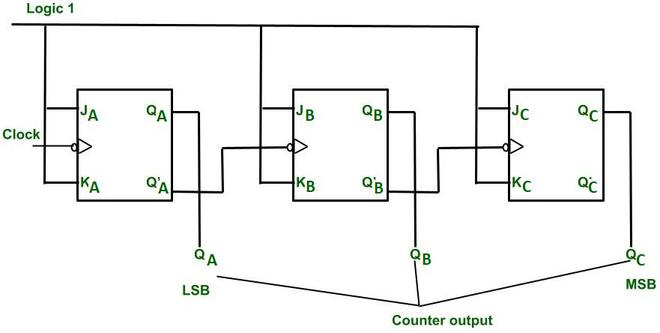By both implementations, we can acquire the same counting states.

Timing diagram –
The working of counter can be easily understood by the timing diagrams.

Explanation –

• Here the -ve edge clock pulse is used(i.e. the counter state transition can occur only at falling edge of the clock pulse) , therefore toggling will take place.
• All the counter states are generated by the frequency division.
• Initially QA =0 QB =0 and QC= 0.

First circuit –

• The -ve edge clock pulse is provided to 1st counter. Therefore, the output state of the first counter(i.e. QA) will be toggled at every falling edge of the clock pulse.
• As the QA is feed as a clock to the second FF, therefore the output state (i.e. QB) will be toggled at every falling edge of QA.
• In the same manner, the QB acts as clock for the third FF, therefore the output state (QC) of the third FF will be toggled for every falling edge of QB.
• As we know, this is the working of UP counter, but here the output is taken as in complemented form (i.e. Q’C Q’B Q’A), therefore we get the complemented outputs(i.e. down counting 111 to 000)
• After the 8th falling edge of the external clock pulse, the counter is reset to 000.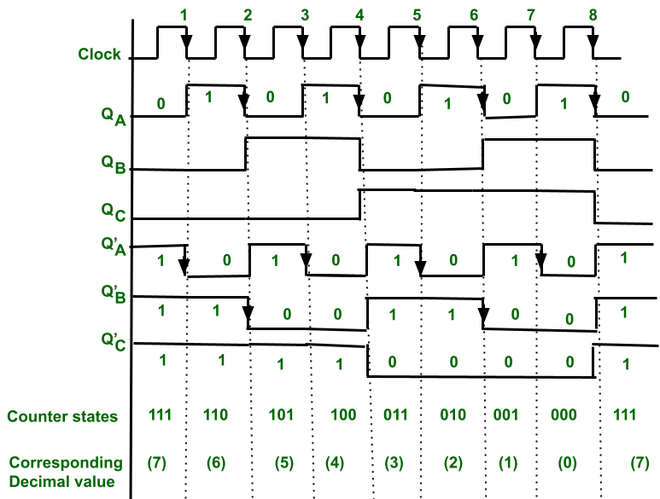Timing diagram for first circuit

Second circuit –

• The -ve edge clock pulse is provided to 1st counter. Therefore, the output state of the first counter(i.e. QA) will be toggled at every falling edge of the clock pulse.
• As the complemented output(Q’A) is feed as a clock to second FF, therefore the output state (i.e. QB) will be toggled at every falling edge of Q’A.
• In the same manner, the Q’B acts as a clock for the third FF, therefore the output state (QC) of the third FF will be toggled for every falling edge of Q’B.
• In this case, the outputs from the three FF are taken as (QC QB QA).
• After the 8th falling edge of the external clock pulse, the counter is reset to 000.
• Here, the down counting states can be acquired after first -ve edge clock pulse(i.e. after first -ve pulse the counting output becomes  111).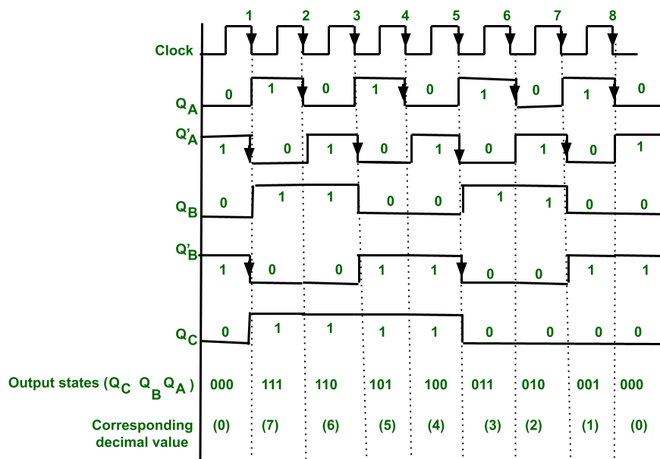Timing diagram for second circuit

• Due to accumulation of propagation delay, they are used in low speed circuits.
• They are simple to design.
• They are used in mod n counters, and in divide by n counter that divides the input by n(i.e. n is integer).

• As the number of flip-flops increases, the propagation delay also increases.
• For high clock frequencies, counting errors may occur because of propagation delay.
For example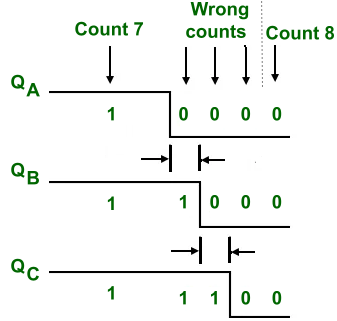Wrong counting state due to propagation delay

Due to propagation delay(by counter), the toggling action takes place after some delay, so by this wrong counting states may be generated.

• In the case of truncated counter (i.e. in mod counters), extra feedback logic(i.e. logic gate) is required.

Attention reader! Don’t stop learning now.  Practice GATE exam well before the actual exam with the subject-wise and overall quizzes available in GATE Test Series Course.

Learn all GATE CS concepts with Free Live Classes on our youtube channel.

My Personal Notes arrow_drop_up